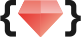# 五倍紅寶石 ・專業程式教育機構

## 等號究竟是什麼意思？ Elixir 裡的 pattern matching

By Tai-An Su・12月 31 2019・技術文章image via Imaiges

``````# Ruby
a, b = [1, 2]
#=> a 是 1， b 是 2``````

``a, a, b = [1, 2, 3]``

``````[a, a, b] = [1, 2, 3]

\#=>  ** (MatchError) no match of right hand side value: [1,2,3]``````

Elixir 告訴你，「同學同學，`a` 不可能同時是 `1` 又是 `2`你的數學老師在你後面，他非常火。

## Pattern Matching: 找個辦法讓等號左右兩邊比對成功

``````[a, a, b] = [1, 1, 3]

\#=> [1, 1, 3]``````

``````[a, 3, b] = [1, 2, 3]

\#=> ** (MatchError) no match of right hand side value: [1,2,3]

[a, 2, b] = [1, 2, 3]

\#=> [1,2,3]``````

### 串列比對與連續比對

``````[head | tails] = [1, 2, 3, 4]

\#=> head 是 1，tails 是 [2, 3, 4]``````

`|` 是串列比對中切開首值及剩下的串列的方式，如同 LISP 中的 `car``cdr`

``````[h | t] = [1, b, c, d] = [1, 2, 3, 4]

\#=> b = 2, c = 3, d = 4
\#=> h = 1, t = [2, 3, 4]``````

### `_`： 我不在乎這個

``````[a, b | _] = [1, 2, 3, 4, 5]

\#=> a = 1, b = 2``````

``````[first, _second, third | _tails] = [1, 2, 3, 4, 5]
#=> first = 1, third = 3
#=> 雖然可以用 _second 及 _tails 拿到值，但是 Elixir 會噴警告給你。``````

### `^`： 將變數求值進行比對

``````a = 0

[^a, b, 2 | _tails] = [0, 1, 2, 3, 4]

\#=> b = 1``````

## 函式的 pattern matching

``````defmodule Shape do
def area({:square, w}), do: w * w
def area({:circle, r}), do: r * r * 3.14
def area({:rectangle, w, h}), do: w * h
end

Shape.area({:square, 5}) #=> 25
Shape.area({:circle, 10}) #=> 314
Shape.area({:rectangle, 5, 10}) #=> 50``````

### 還可以多加一點判斷

``````defmodule Alcohol do
def check_age(_, age) when age < 18, do: "你年紀太小囉"
def check_age(name, _), do: "歡迎，#{name}"
end``````

``````defmodule Fibonacci do
def get(1) when n == 0 or n == 1, do: n
def get(n), do: get(n - 1) + get(n - 2)
def list(n), do: for x <- 0..n - 1, do: get(x)
end``````

## 小結

Pattern matching 在 Elixir (及許多函數式編程語言，如 Haskell) 中扮演了非常重要的角色。可以讓你寫出非常簡潔而且易懂的程式，而且會讓你開始習慣用找出邊界情況遞迴的角度，來思考如何組織程式。

## 工商服務Happy hacking! 下次見。

## 幫助與政策

• ### 服務條款

© 2014 - 2023 五倍紅寶石程式資訊教育股份有限公司 (5xRuby Training Group CO., LTD) | 台北市短期補習班立案 第 7594 號